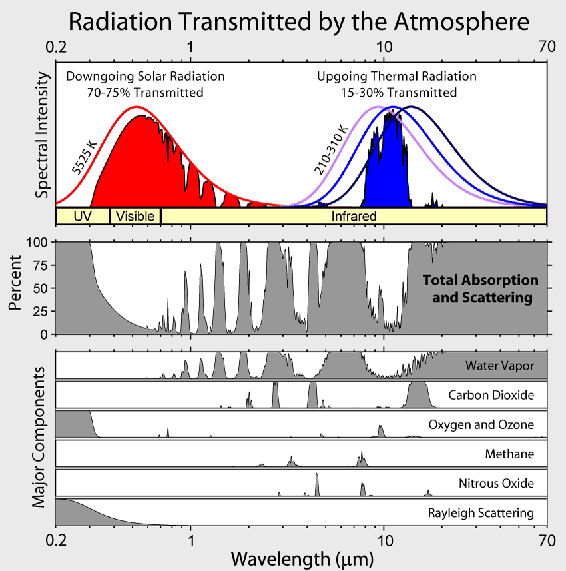## Saturday, 5 August 2017

### Greenhouse gas effect (GHGE) is overwhelmingly caused by water vapour. Not carbon dioxide.

Claim: The greenhouse gas effect (GHGE) is overwhelmingly caused by water vapour. Not carbon dioxide.

The current "consensus" says: the GHGE is very important at the equator (so climate models say). Approximately between +20º and -20º latitude. This extra heat 'trapped there' rises and settles down at the poles thereby warming the planet. This extra trapped heat is due to more CO2 from burning fossil fuels. Models say 24% of the GHGE is due to CO2. That about 72% is due to water and ~ 4% to other gases (mostly Ozone).

Let us move away from assumptions and models (alarmism) to basic science (below), which no one can dispute:

1. The sun angle controls water vapour content of the atmosphere. So the atmosphere in the equatorial zone is 80× to 90× more H2O than CO2. [see diagram below]
2. Water is a much more powerful GHG than CO2 because the bonds in water have far more degrees of freedom so can undergo far more electron bond transitions. It's these bond transitions which cause infrared (IR) radiation absorption. CO2 can only absorb IR at 3 frequencies. Water absorbs at dozens of frequencies.
3. A plot of GHG forcing against water vapour in the atmosphere shows "the upper limit to the back radiation of GHGs is approximately 420 W/m² at water vapour concentration of approximately 32,000 ppmv, or an H2O/CO2 ratio of 80:1; (2) the lowest back radiation is 97 W/m² at the South Pole" where the ratio H2O:CO2 is 1:1.#### This Is The Clincher:

Consider at the South pole where CO2:H2O is 1:1 and GHGE = 97W/m². If that is 24% due to CO2, it means CO2 effect = 23W/m² (at the poles). Moving on to the equator: CO2 is well-mixed so it's also 23W/m² out of 420 W/m² there. That means only 5.5% of GHGE at equator (where it really matters) is due to CO2.

The GHGE is said to be responsible for warming the climate by 33ºC above what it would be with no GHGE. From -18ºC to +15ºC. (Averaged). The US state of North Carolina has an average temperature of about 15.3ºC. NC is located latitude 33° 50' N to 36° 35' N. On Average that's 34.2 °N Let's treat it as the average place on Earth. Assume the ratio of H2O to CO2 = 34:1 there. Implying GHGE ~ 345 W/m². Subtract the part due to CO2 then it is 345 -23 = 322 W/m² due to H2O and O3.

### What's the maximum CO2 GHGE?

Let us do a basic maths back of the envelope calculation to give us a maximum for the CO2 GHGE. It can not be more than half of the polar effect. Because even at the poles there's as much water in the atmosphere as CO2, and water is a more powerful GHG [see the last diagram below for a comparison of water and carbon dioxide GHGE]. Let's say the upper limit of the CO2 GHGE is 33% (at the poles) = 33 W/m². Let's count squares in the diagram above. 7 × 33 ÷ 50 = 4.62 squares show CO2 GHGE. The diagram has ~ 49 squares. 4.62 ÷ 49 = 9.5%. That puts an upper limit on the CO2 GHGE of 9.5%. 9.5% of 33ºC = 3.1ºC. (given earth's surface is 33ºC warmer than it would be with not GHGE.)

Remember how the effect of more CO2 tails off logarithmically?So the CO2 GHGE is already nearly maxed out at about 3.1ºC, and most of that was due to the first 20ppm of CO2 in that atmosphere. Doubling CO2, from 280ppm to 560ppm does not double the effect. It only increases by a fraction of a degree.